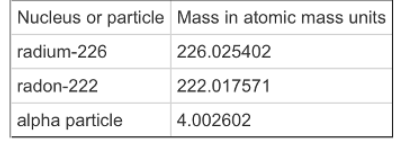# Problem: Part AAn isotope of potassium with half-life of roughly one billion years is often used in radioactive dating. This isotope decays through electron capture, the process of drawing an electron into the nucleus. What is the chemical symbol for the element form this process? The equation for the reaction is 4019K + 0-1e → ?Part BThe reaction energy of a particular reaction is the amount of energy released by the reaction. It is found by determining the differences in mass between the reactants and products and then use E=mc2 to convert the lost mass into the released energy. Look again at the equation for the decay of radium (Ra), given in the introduction (22286Rn → AZX + 42He). Use the following table of masses to determine the reaction energy of this reaction.

###### FREE Expert Solution

Part A

The equation for the reaction is:

4019K + 0-1e → 4018X

97% (342 ratings)###### Problem Details

Part A

An isotope of potassium with half-life of roughly one billion years is often used in radioactive dating. This isotope decays through electron capture, the process of drawing an electron into the nucleus. What is the chemical symbol for the element form this process? The equation for the reaction is

4019K + 0-1e → ?

Part B

The reaction energy of a particular reaction is the amount of energy released by the reaction. It is found by determining the differences in mass between the reactants and products and then use E=mc2 to convert the lost mass into the released energy.

Look again at the equation for the decay of radium (Ra), given in the introduction (22286Rn → AZX + 42He). Use the following table of masses to determine the reaction energy of this reaction.